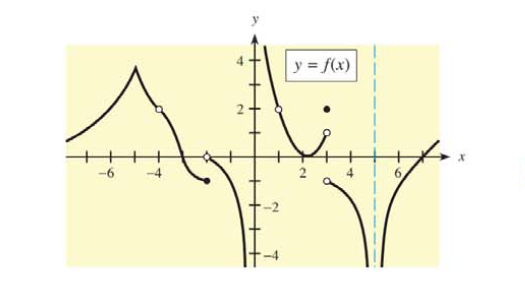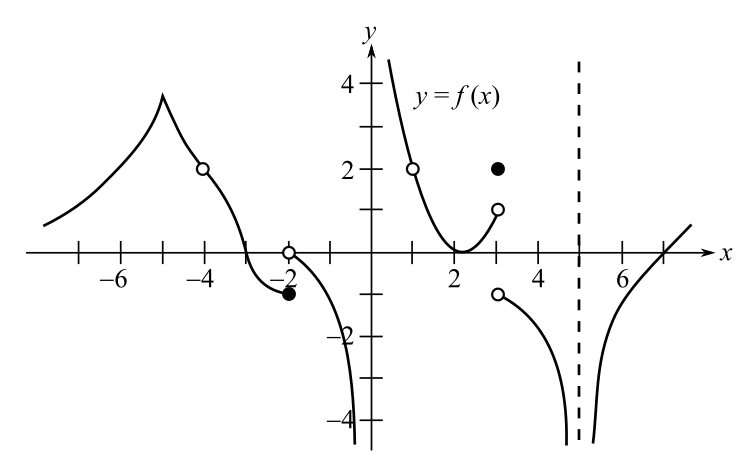Chapter 9.2, Problem 1EMathematical Applications for the ...

12th Edition
Ronald J. Harshbarger + 1 other
ISBN: 9781337625340

Solutions

Chapter
SectionMathematical Applications for the ...

12th Edition
Ronald J. Harshbarger + 1 other
ISBN: 9781337625340
Textbook Problem

In Problems 1 and 2, refer to the figure. For each given x-value, use the figure to determine whether the function is continuous or discontinuous at that x-value. If the function is discontinuous, state which of the three conditions that define continuity is not satisfied.(a) x = − 5 (b) x = 1 (c) x = 3 (d) x = 0

(a)

To determine

Whether the function y=f(x) is continuous or discontinuous and the conditions used to reach the inference from the figure when the point of evaluation is x=5.Explanation

Given Information:

The graph is given as

Explanation:

For a function f(x) to be continuous at a point k, it must satisfy the following conditions:

The value of a function f(x) at the point k must exist.

The limit as xk for the function f(x) must exist.

The value of a function f(x) at the point k and the limit as xk for the function f(x) must be equal.

The graph is given as;

From the graph, the value of the function f(x) at x=5 is;

f(5)=4

For the limit as x5 to exist, the left-side limit must be equal to the right-hand limit

(b)

To determine

Whether function y=f(x) is continuous or discontinuous and the conditions used to reach the inference from the figure when the point of evaluation is x=1.(c)

To determine

Whether function y=f(x) is continuous or discontinuous and the conditions used to reach the inference from the figure when the point of evaluation is x=3.(d)

To determine

Whether function y=f(x) is continuous or discontinuous and the conditions used to reach the inference from the figure when the point of evaluation is x=0.Still sussing out bartleby?

Check out a sample textbook solution.

See a sample solution

The Solution to Your Study Problems

Bartleby provides explanations to thousands of textbook problems written by our experts, many with advanced degrees!

Get Started

Describe the set of points (x,y) such that x2+y2=0.

Finite Mathematics and Applied Calculus (MindTap Course List)

Given: mFMH=4x+1 m4=x+4 Find: m4

Elementary Geometry for College Students

Evaluate 6 8 24 30

Study Guide for Stewart's Multivariable Calculus, 8th